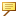Specifying Default Dimensional Tolerances
When you begin creating a model, Creo Parametric uses default dimensional tolerance values. Therefore, you must specify default tolerances beforehand by setting the configuration file options linear_tol and angular_tol. Using linear_tol, you can set default tolerances for integer dimensions as well.
A linear dimensional tolerance does not have to have the same number of decimal places as the dimension. That is, dimensions can acquire tolerances that have a higher significant number of decimal places (num digits).
For example, a dimension with six decimal places can have a tolerance value with seven decimal places. To set the default display of linear dimensional tolerances, specify the value of linear_tol as follows:
# tolerance x
- where # is an integer representing the number of decimal digits for the dimension, tolerance represents the default tolerance value, and x specifies the number of decimal digits for the tolerance.
The x value must be equal to or greater than the # value. If x is less than #, the system uses the default value (equal to the number of decimal digits for the dimension) and rounds it to the significant number of digits of the dimension.If you do not include x in the value, the system sets the default display equal to the number of decimal digits of the dimension.
Example
Linear_tol 0 .05 2
For the tolerances specified in the example, a dimension of a whole number would have a tolerance of .05.
When you begin creating a part, the system displays a table at the bottom of the window with the current default tolerances. This table appears only when you have set the configuration file option tol_display to yes.
If you have not specified tolerances, Creo Parametric assumes the system defaults.